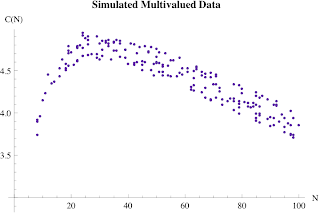## Wednesday, August 27, 2008

### Applying the USL Model to Multivalued Data

A reader was having trouble applying the USL model to his data and asked me to take a look at it. I can't discuss his data because it's private, but I can show you what I did using some simulated data that has the same properties. The data was collected off a production system not a controlled load-test platform, and this brought to the surface two aspects of applying the USL which I had not faced previously:

1. The data is in the form of sampled time series. Instead of throughput X(N) as a function of N users, the data is in the form X(t) and N(t) as functions of time t.
2. The data is multi-valued. Within each sample period there were multiple user loads with the same value but different throughput values. For example:
 Hr X(t) N(t) 7 12 1 7 12 1 8 166 22 8 462 69 8 680 149 9 282 45 9 310 55 9 291 55
Fresh from the Guerrilla Data Analysis Techniques class, I decided to give it a shot. The times-series problem (i.e., data ordered by time) can be addressed by simply ranking the data according to the user load (N) rather than by time (t). Since the measurement intervals are of 1 hour duration, we will assume that those data reach steady state to a reasonable approximation. This may not always be valid, so we have to keep it in mind. Next comes the multivaluedness. In the above sample data, you can see that hour-9 has two samples with the same user load i.e., N = 55, but different throughput values. Nonetheless, we press on and normalize all the data to the N = 1 value X(1) = 12 in hour-7. That allows us to calculate the relative capacity function C(N) = X(N)/X(1). Here's that looks like using simulated data:The question is, can we fit the USL nonlinear model to this multi-valued data? The answer is yes, because the regression algorithms shouldn't care. After all, that's generally what raw data looks like. Here's how it looks for the simulated data:The solid curve is produced by the USL model. I used Mathematica, but it should work with any regression package. Typically, I never see data in this form because there is usually only a single throughput value X(N) for each user-load value (N) in a controlled test environment. Chalk up another one to USL, and thanks to the reader for raising the question.